# PSEB 11th Class Physics Solutions Chapter 8 Gravitation

Punjab State Board PSEB 11th Class Physics Book Solutions Chapter 8 Gravitation Textbook Exercise Questions and Answers.

## PSEB Solutions for Class 11 Physics Chapter 8 Gravitation

PSEB 11th Class Physics Guide Gravitation Textbook Questions and Answers

Question 1.
(a) You can shield a charge from electrical forces by putting it inside a hollow conductor. Can you shield a body from the gravitational influence of nearby matter by putting it inside a hollow sphere or by some other means?
(b) An astronaut inside a small spaceship orbiting around the earth cannot detect gravity. If the space station orbiting around the earth has a large size, can he hope to detect gravity?
(c) If you compare the gravitational force on the earth due to the sun to that due to the moon, you would find that the Sun’s pull is greater than the moon’s pull. (You can check this yourself using the data available in the succeeding exercises). However, the tidal effect of the moon’s pull is greater than the tidal effect of sun. Why?
(a) No, Gravitational influence of matter on nearby objects cannot be screened by any means. This is because gravitational force unlike electrical forces is independent of the nature of the material medium. Also, it is independent of the status of other objects.

(b) Yes, If the size of the space station is large enough, then the astronaut will detect the change in Earth’s gravity (g).

(c) Tindal effect depends inversely upon the cube of the distance while gravitational force depends inversely on the square of the distance. Since the distance between the Moon and the Earth is smaller than the distance between the Sun and the Earth, the tidal effect of the Moon’s pull is greater than the tidal effect of the Sun’s pull.

Question 2.
Choose the correct alternative:
(a) Acceleration due to gravity increases/decreases with increasing altitude.
(b) Acceleration due to gravity increases/decreases with increasing depth (assume the earth to be a sphere of uniform density).
(c) Acceleration due to gravity is independent of mass of the earth/mass of the body.
(d) The formula -G Mm(1 /r2 -1/r1) is more/less accurate than the formula mg (r2 – r1) for the difference of potential energy between two points r2 and r1 distance away from the centre of the earth.
Solution:
(a) Decreases,
Explanation : Acceleration due to gravity at altitude h is given by the relation:
gh = $$\left(1-\frac{2 h}{R_{e}}\right) g$$
where, Re = Radius of the Earth
g = Acceleration due to gravity on the surface of the Earth
It is clear from the given relation that acceleration due to gravity decreases with an increase in height.

(b) Decreases,
Explanation : Acceleration due to gravity at depth d is given by the relation:
gd = $$\left(1-\frac{d}{R_{e}}\right) g$$
It is clear from the given relation that acceleration due to gravity decreases with an increase in depth.

(c) Mass of the body,
Explanation : Acceleration due to gravity of body of mass m is given by the relation:
g = $$\frac{G M_{e}}{R^{2}}$$
where, G = Universal gravitational constant
Me = Mass of the Earth
Re = Radius of the Earth
Hence, it can be inferred that acceleration due to gravity is independent of the mass of the body.

(d) More,
Explanation : Gravitational potential energy of two points r2 and r1 distance away from the centre of the Earth is respectively given by:
V(r1) = -GmM
V(r2) = – $$\frac{G m M}{r_{2}}$$
V = V (r2) – V(r1) = -GmM$$\left(\frac{1}{r_{2}}-\frac{1}{r_{1}}\right)$$.
Hence, this formula is more accurate than the formula mg (r2 – r1).

Question 3.
Suppose there existed a planet that went around the Sun twice as fast as the Earth. What would be its orbital size as compared to that of the Earth?
Solution:
Time taken by the Earth to complete one revolution around the Sun,
Te = 1 year
Orbital radius of the Earth in its orbit, Re = 1 AU
Time taken by the planet to complete one revolution around the Sun,
Tp = $$\frac{1}{2} T_{e}=\frac{1}{2}$$ year
Orbital radius of the planet = Rp
From Kepler’s third law of planetary motion, we can write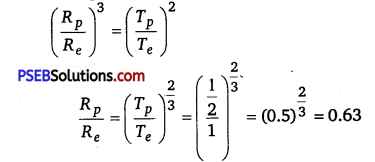Hence, the orbital radius of the planet will be 0.63 times smaller than that of the Earth.

Question 4.
I0, one of the satellites of Jupiter, has an orbital period of 1.769 days and the radius of the orbit is 4.22 x 108 m. Show that the mass of Jupiter is about one-thousandth that of the sun.
Solution:
Orbital period of I0, TI0 = 1.769 days = 1.769 x 24 x 60 x 60 s
Orbital radius of II0, RI0 = 4.22 x 108 m
Satellite I0 is revolving around the Jupiter Mass of the latter is given by the relation:
Mj = $$\frac{4 \pi^{2} R_{I_{O}}^{3}}{G T_{I_{O}}^{2}}$$ ……………………….. (i)

where Mj = Mass of Jupiter
G = Universal gravitational constant Orbital period of the Earth,
Te = 365.25 days = 365.25 x 24 x 60 x 60 s Orbital radius of the Earth,
Re =1 AU = 1.496 x 1011 m
Mass of Sun is given as:
Ms = $$\frac{4 \pi^{2} R_{e}^{3}}{G T_{e}^{2}}$$ ………………………. (ii)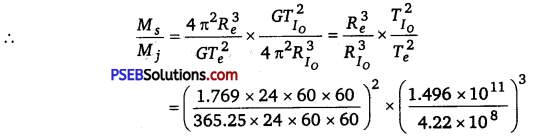= 1045.04
∴ $$\frac{M_{s}}{M_{j}} \sim 1000$$
$$M_{s} \sim 1000 \times M_{j}$$
Hence, it can be inferred that the mass of Jupiter is about one thousandth that of the Sun.

Question 5.
Let us assume that our only consists of 2.5 x 1011 stars each of one solar mass. How long will a star at a distance of 50,000 ly from the galactic center take to complete one revolution? Take the diameter of the Milky Way to be 105 ly.
Solution:
Number of stars in our galaxy, = 2.5 x 1011
Mass of each star = one solar mass =2 x 1030 kg
Mass of our galaxy,M =2.5 x 1011 x 2 x 1030 = 5 x 1041 kg
Diameter of Milky Way, d = 105 ly
Radius of Milky Way,r= 5 x 104 ly
∵ 1 ly=946 x 1015m
∴ r=5 x 104 x 9.46 x 1015
=4.73 x 1020m

Since a star revolves around the galactic centre of the Milky Way, its time period is given by the relation:
T = $$\left(\frac{4 \pi^{2} r^{3}}{G M}\right)^{1 / 2}$$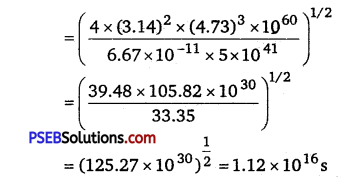1 year = 365 x 324 x 60 x 60 s
∵ 1 s = $$\frac{1}{365 \times 24 \times 60 \times 60}$$ years
∴ 1.12 x 1016 s = $$\frac{1.12 \times 10^{16}}{365 \times 24 \times 60 \times 60}$$ = 3.55 x 10 8 years

Question 6.
Choose the correct alternative:
(a) If the zero of potential energy Is at infinity, the total energy of an orbiting satellite is negative of its kinetic/potential energy.
(b) The energy required to launch an orbiting satellite out of Earth’s gravitational influence Is more/less than the energy required to project a stationary object at the same height (as the satellite) out of Earth’s influence.
Solution:
(a) Kinetic energy
Explanation: Total mechanical energy of a satellite is the sum of its kinetic energy (always positive) and potential energy (maybe negative). At infinity, the gravitational potential energy of the satellite is zero. As the Earth-satellite system is a bound system, the total energy of the satellite is negative.

Thus, the total energy of an orbiting satellite at infinity is equal to the negative of its kinetic energy.

(b) Less
Explanation: An orbiting satellite acquires certain amount of energy that enables it to revolve around the Earth. This energy is provided by its orbit. It requires relatively lesser energy to move out of the influence of the Earth’s gravitational field than a stationary object on the Earth’s surface that initially contains no energy.

Question 7.
Does the escape speed of a body from the Earth depend on
(a) the mass of the body,
(b) the location from where it is projected,
(c) the direction of projection,
(d) the height of the location from where the body is launched?
(a) No, we know that the escape velocity of the body is given by
Ve = $$\sqrt{\frac{2 G M}{R}}$$ , where M and R are mass and radius of Earth. Thus clearly, it does not depend on the grass of the body as Ve is independent of it.

(b) Yes, we know that Ve depends upon the gravitational potential at the point from where the body is launched. Since the gravitational potential depends on the latitude and height of the point, therefore the escape velocity depends on the location of the point from where it is projected. It can also be experienced as:
Ve = $$\sqrt{2 g r}$$ . As g has different values at different heights. Therefore, Ve depends upon the height of location.

(c) No, it does not depend on the direction of projection as Ve is independent of the direction of projection.

(d) Yes, it depends on the height of location from where the body is launched as explained in (b).

Question 8.
A comet orbits the Sun in a highly elliptical orbit. Does the comet have a constant
(a) linear speed,
(b) angular speed,
(c) angular momentum,
(d) kinetic energy,
(e) potential energy,
(f) total energy throughout its orbit? Neglect any mass loss of the comet when it comes very close to the Sun.
Solution:
(a) No, according to law of conservation of linear momentum L = mvr constant, therefore the comet moves faster when it is close to the sun and moves slower when it is farther away from the sun. Therefore, the speed of the comet does not remain constant.

(b) No, as the linear speed varies, the angular speed also varies. Therefore, angular speed of the comet does not remain constant.

(c) Yes, as no external torque is acting on the comet, therefore, according to law of conservation of angular momentum, the angular momentum of the comet remain constant.

(d) No, kinetic energy of the comet = $$\frac{1}{2}$$ mν2 As the linear speed of the comet changes as its kinetic energy also changes. Therefore, its KE does not remains constant.

(e) No, potential energy of the comet changes as its kinetic energy changes.

(f) Yes, total energy of a comet remain constant throughout its orbit.

Question 9.
Which of the following symptoms is likely to afflict an astronaut in space
(a) swollen feet,
(b) swollen face,
(d) orientational problem?
(b), (c), and (d)
Explanations:
(a) Legs hold the entire mass of a body in standing position due to gravitational pull. In space, an astronaut feels weightlessness because of the absence of gravity. Therefore, swollen feet of an astronaut do not affect him/her in space.

(b) A swollen face is caused generally because of apparent weightlessness in space sense organs such as eyes, ears, nose, and mouth constitute a person’s face. This symptom can affect an astronaut in space.

(c) Headaches are caused because of mental strain. It can affect the working of an astronaut in space.

(d) Space has different orientations. Therefore, orientational problems can affect an astronaut in space.

Question 10.
In the following two exercises, choose the correct answer from among the given ones:
The gravitational intensity at the center of a hemispherical shell of uniform mass density has the direction indicated by the arrow (see figure) (i) a, (ii) b, (iii) c, (iv) 0.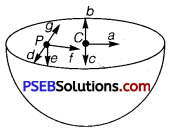Solution:
(iii) Gravitational potential (V) is constant at all points in a spherical shell.
Hence, the gravitational potential gradient $$\left(\frac{d V}{d r}\right)$$ is zero everywhere inside the spherical shell. The gravitational potential gradient is equal to the negative of gravitational intensity. Hence, intensity is also zero at all points inside the spherical shell. This indicates that gravitational forces acting at a point in a spherical shell are symmetric.

If the upper half of a spherical shell is cut out (as shown in the given figure), then the net gravitational force acting on a particle located at center O will be in the downward direction.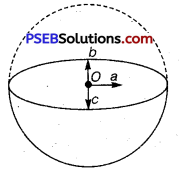Since gravitational intensity at a point is defined as the gravitational force per unit mass at that point, it will also act in the downward direction. Thus, the gravitational intensity at center O of the given hemispherical shell has the direction as indicated by arrow c.

Question 11.
For the above problem, the direction of the gravitational intensity at an arbitrary point P is indicated by the arrow
(i) d,
(ii) e,
(iii) f,
(iv) g.
Solution:
(ii) Gravitational potential (V) is constant at all points in a spherical shell.
Hence, the gravitational potential gradient $$\left(\frac{d V}{d r}\right)$$ inside the spherical shell. The gravitational potential gradient is equal to the negative of gravitational intensity. Hence, intensity is also zero at all points inside the spherical shell. Ibis indicates that gravitational forces acting at a point in a spherical shell are symmetric. If the upper half of a spherical shell is cut out (as shown in the given figure), then the net gravitational force acting on a particle at an arbitrary point P will be in the downward direction.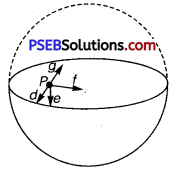Since gravitational intensity at a point is defined as the gravitational force per unit mass at that point, it will also act in the downward direction. Thus, the gravitational intensity at an arbitrary point P of the hemispherical shell has the direction as indicated by arrow e.

Question 12.
A rocket Is fired from the Earth towards the Sun. At what distance from the Earth’s centre is the gravitational force on the rocket zero? Mass of the Sun = 2 x 1030kg, mass of the Earth = 6 x 1024 kg. Neglect the effect of other planets etc. (orbital radius = 1.5 x 1011 m)
Solution:
Mass of the Sun, Ms = 2 x 1030 kg
Mass of the Earth, Me = 6 x 10 24 kg
Orbital radius, r = 1.5 x 1011 m
Mass of the rocket = mLet x be the distance from the center of the Earth where the gravitational force acting on satellite P becomes zero.
From Newton’s law of gravitation, we can equate gravitational forces acting on satellite P under the influence of the Sun and the Earth as: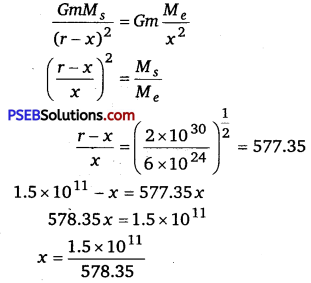= 2.59 x 108 m

Question 13.
How will you ‘weigh the Sun’, that is estimate its mass? The mean orbital radius of the Earth around the Sun is 1.5 x 108 km.
Solution:
Orbital radius of the Earth around the Sun, r = 1.5 x 108 km = 1.5 x 1011 m
Time taken by the Earth to complete one revolution around the Sun,
T = 1 year = 365.25 days = 365.25 x 24 x 60 x 60 s
Universal gravitational constant, G = 6.67 x 10-11N-m2 kg-2
Thus, mass of the Sun can be calculated using the relation,
M = $$\frac{4 \pi^{2} r^{3}}{G T^{2}}$$
= $$\frac{4 \times(3.14)^{2} \times\left(1.5 \times 10^{11}\right)^{3}}{6.67 \times 10^{-11} \times(365.25 \times 24 \times 60 \times 60)^{2}}$$
= $$\frac{133.24 \times 10^{33}}{6.64 \times 10^{4}}$$ = 2.0 x 1030kg
Hence, the mass of the Sun is 2.0 x 1030 kg.

Question 14.
A Saáurn year is 29.5 times the Earth year. How far is the Saturn from the Sun if the Earth is 1.5 x 108 km away from the Sun?
Solution:
Distance of the Earth from the Sun, re = 1.5x 108 km= 1.5 x 1011 m
Time period of the Earth = Te
Time period of Saturn, Ts = 29.5 Te
Distance of Saturn from the Sun = rs
From Kepler’s third law of planetary motion, we have
T=$$\left(\frac{4 \pi^{2} r^{3}}{G M}\right)^{\frac{1}{2}}$$
For Saturn and Sun, we can write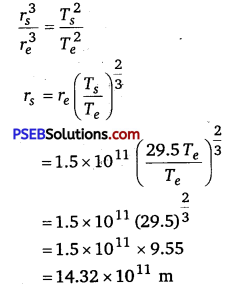= 1.43 x 1012 m

Question 15.
A body weigths 63 N on the surface of the Earth. What is the gravitational force on it due to the Earth at a height equal to half the radius of the Earth?
Solution:
Weight of the body, W =63 N
Acceleration due to gravity at height h from the Earth’s surface is given by the relation:
g’ = $$\frac{g}{\left(1+\frac{h}{R_{e}}\right)^{2}}$$
where, g = Acceleration due to gravity on the Earth’s surface
For h= $$\frac{R_{e}}{2}$$
g’ = $$\frac{g}{\left(1+\frac{R_{e}}{2 \times R_{e}}\right)^{2}}=\frac{g}{\left(1+\frac{1}{2}\right)^{2}}=\frac{4}{9} g$$

Weight of a body of mass m at height h is given as
W’ = mg’
= m x $$\frac{4}{9}$$ g = $$\frac{4}{9}$$ x mg
= $$\frac{4}{9}$$ W
= $$\frac{4}{9}$$ x 63 =28 N

Question 16.
Assuming the Earth to be a sphere of uniform mass density, how much would a body weigh halfway down to the centre of the Earth if it weighed 250 N on the surface?
Solution:
Weight of a body of mass m at the Earth’s surface, W = mg = 250 N
Body of mass m is located at depth, d = $$\frac{1}{2}$$ Re
where, Re = Radius of the Earth
Acceleration due to gravity at depth d is given by the relation
g’ = $$\left(1-\frac{d}{R_{e}}\right) g=\left(1-\frac{R_{e}}{2 \times R_{e}}\right) g=\frac{1}{2} g$$
Weight of the body at depth d,
W’ = mg’
= m x $$\frac{1}{2} g=\frac{1}{2}$$ mg = $$\frac{1}{2}$$ W
= $$\frac{1}{2}$$ x 250 = 125 N

Question 17.
A rocket is fired vertically with a speed of 5 km s-1 from the Earth’s surface. How far from the Earth does the rocket ‘ go before returning to the earth? Mass of the Earth = 6.0 x 1024 kg; mean radius of the Earth = 6.4 x 106 m;
G = 6.67 x 10 -11 N-m2 kg-2.
Solution:
Velocity of the rocket, ν = 5 km/s = 5 x 103 m/s
Mass of the Earth, Me = 6.0 x 1024 kg
Radius of the Earth, Re = 6.4 x 106 m
Height reached by rocket mass, m = h

At the surface of the Earth,
Total energy of the rocket = Kinetic energy + Potential energy
= $$\frac{1}{2} m v^{2}+\left(\frac{-G M_{e} m}{R_{e}}\right)$$
At highest point h,
ν = 0
and, Potential energy = – $$\frac{G M_{e} m}{R_{e}+h}$$
Total energy of the rocket = 0+ $$\left(-\frac{G M_{e} m}{R_{e}+h}\right)=-\frac{G M_{e} m}{R_{e}+h}$$
From the law of conservation of energy, we have
Total energy of the rocket at the Earth’s surface=Total energy at height h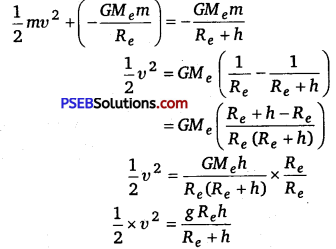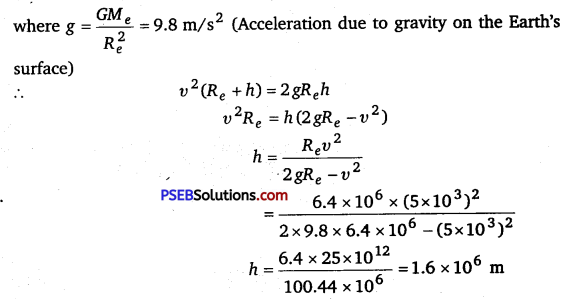Height achieved by the rocket with respect to the centre of the Earth = Re +h
= 6.4 x 106 +1.6 x 106 = 8.0 x 106 m

Question 18.
The escape speed of a projectile on the Earth’s surface is 11.2 km s-1. A body is projected out with thrice this speed. What is the speed of the body far away from the Earth? Ignore the presence of the Sun and other planets.
Solution:
Escape velocity of a projectile from the Earth, υesc = 11.2 km/s
Projection velocity of the projectile, vp = 3 vesc
Mass of the projectile = m
Velocity of the projectile far away from the Earth = vf
Total energy of the projectile on the Earth = $$\frac{1}{2} m v_{p}^{2}-\frac{1}{2} m v_{\mathrm{esc}}^{2}$$
The gravitational potential energy of the projectile far away from the Earth is zero.
Total energy of the projectile far away from the Earth = $$\frac{1}{2}$$ mv2f
From the law of conservation of energy, we have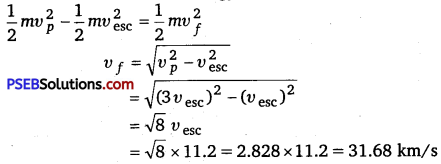Question 19.
A satellite orbits the Earth at a height of 400 km above the surface. How much energy must be expended to rocket the satellite out Of the Earth’s gravitational influence? Mass of the satellite = 200 kg mass of the Earth = 6.0 x 1024 kg; radius of the Earth=6.4x 106 m;G=6.67 x 10-11 N-m2 kg-2.
Solution:
Mass of the Earth, Me =6.0 x 1024 kg
Mass of the satellite, m = 200 kg
Radius of the Earth, Re = 6.4 x 106 m
Universal gravitational constant, G = 6.67 x 10-11 N-m2kg2
Heightofthesatellite,h =400 km=4 x 105 m=0.4 x 106 m

Total energy of the satellite at height h = $$\frac{1}{2} m v^{2}+\left(\frac{-G M_{e} m}{R_{e}+h}\right)$$
Orbital velocity of the satellite, ν = $$\sqrt{\frac{G M_{e}}{R_{e}+h}}$$
Total energy of height, h = $$\frac{1}{2} m\left(\frac{G M_{e}}{R_{e}+h}\right)-\frac{G M_{e} m}{R_{e}+h}=-\frac{1}{2}\left(\frac{G M_{e} m}{R_{e}+h}\right)$$

The negative sign indicates that the satellite is bound to the Earth. This is called bound energy of the satellite.
Energy required to send the satellite out of its orbit = – (Bound energy)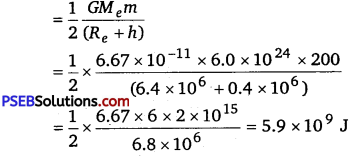Question 20.
Two stars each of one solar mass (= 2x 1030 kg) are approaching each other for a head-on collision. When they are a distance 109 km, their speeds are negligible. What is the speed with which they collide? The radius of each star is 104 km. Assume the stars to remain undistorted until they collide. (Use the known value of G).
Solution:
Mass of each star, M = 2 x 1030 kg
Radius of each star, R = 104 km = 107 m
Distance between the stars, r = 109 km = 1012 m
For negligible speeds, ν = 0 total energy of two stars separated at distance r
= $$\frac{-G M M}{r}+\frac{1}{2} m v^{2}$$
= $$\frac{-G M M}{r}+0$$ = 0 ………………………… (i)

Now, consider the case when the stars are about to collide:
The velocity of the stars = ν
Distance between the centers of the stars = 2R
Total kinetic energy of both stars = $$\frac{1}{2} M v^{2}+\frac{1}{2} M v^{2}$$ = Mv2
Total potential energy of both stars = $$\frac{-G M M}{2 R}$$
Total energy of the two stars = Mv2 – $$\frac{-G M M}{2 R}$$ ………………………. (ii)
Using the law of conservation of energy, we can write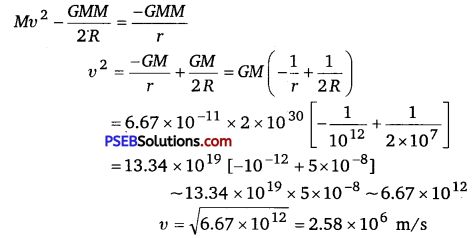Question 21.
Two heavy spheres each of mass 100 kg and radius 0.10 m are placed 1.0 m apart on a horizontal table. What is the gravitational force and potential at the midpoint of the line joining the centers of the spheres? Is an object placed at that point in equilibrium? If so, is the equilibrium stable or unstable?
Solution:
The situation is represented in the following figure :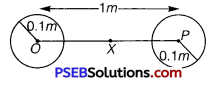Mass of each sphere, M = 100 kg
Separation between the spheres, r = 1 m
X is the mid-point between the spheres. Gravitational force at point X will be zero. This is because gravitational force exerted by each sphere will act in opposite directions. Gravitational potential at point X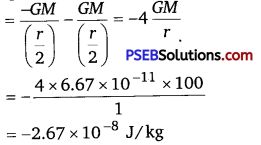Any object placed at point X will be in equilibrium state, but the equilibrium is unstable. This is because any change in the position of the object will change the effective force in that direction.

Question 22.
As you have learnt in the text, a geostationary satellite orbits the Earth at a height of nearly 36,000 km from the surface of the Earth. What is the potential due to Earth’s gravity at the site of this satellite? (Take the potential energy at infinity to be zero). Mass of the Earth = 6.0 x 1024 kg, radius = 6400 km.
Solution:
Mass of the Earth, M = 6.0 x 1024 kg
Radius of the Earth, R = 6400 km = 6.4 x 106 m
Height of a geostationary satellite from the surface of the Earth,
h = 36000 km = 3.6 x 107m
Gravitational potential energy due to Earth’s gravity at height h,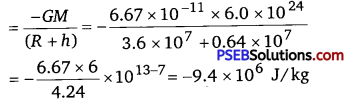Question 23.
A star 2.5 times the mass of the Sun and collapsed to a size of 12 km rotates with a speed of 1.2 revs. per second. (Extremely compact stars of this kind are known as neutron stars. Certain stellar objects called pulsars belong to this category). Will an object placed on its equator remain stuck to its surface due to gravity? (Mass of the Sun = 2 x 1030 kg).
Solution:
Yes, A body gets stuck to the surface of a star if the inward gravitational force is greater than the outward centrifugal force caused by the rotation of the star.
Gravitational force, fg = $$\frac{G M m}{R^{2}}$$
where,M =Mass of the star=2.5 x 2 x 1030 = 5 x 1030 kg
m = Mass of the body

∴ fg = $$\frac{6.67 \times 10^{-11} \times 5 \times 10^{30} \times m}{\left(1.2 \times 10^{4}\right)^{2}}$$
= 2.31 x 1012 mN
Centrifugal force, fc = mrω2

where, ω = Angular speed = 2 πv
ν = Angular frequency = 1.2 rev s-1
f c=mR(2πv)2
= m x (1.2 x 104) x 4 x(3.14)2 x (1.2)2 = 1.7 x 105 mN
Since fg > fc, the body will remain stuck to the surface of the star.

Question 24.
A spaceship is stationed on Mars. How much energy must be expended on the spaceship to launch it out of the solar system? Mass of the spaceship = 1000 kg; mass of the Sun = 2 x 1030 kg; mass of mars = 6.4 x 1023 kg; radius of mars = 3395 km; radius of the orbit of mars = 2.28 x 108 km;
G = 6.67 x 10-11 N-m2kg-2.
Solution:
Given, mass of the Sun, M = 2 x 1030 kg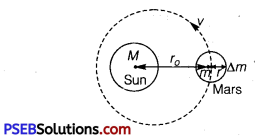Mass of the mars, m = 6.4 x 1023 kg
Mass of spaceship, Δm = 1000 kg
Radius of orbit of the mars, r0 = 2.28 x 1011
Radius of the mars, r = 3.395 x 106 m
If v is the orbital velocity of mars, then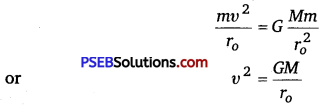Since the velocity of the spaceship is the same as that of the mars, Kinetic energy of the spaceship,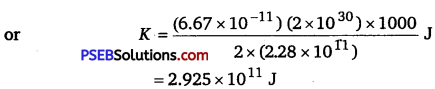Total potential energy of the spaceship,
U = Potential energy of the spaceship due to its being in the gravitational
field of the mars + potential energy of the spaceship due to its being in the gravitational field of the Sun.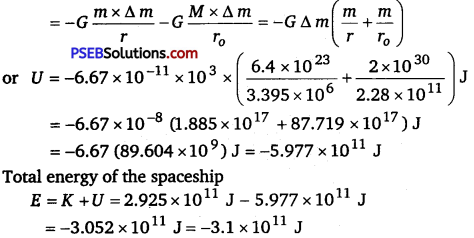Total energy of the spaceship E=K +U = 2.925 x 1011 J – 5.977 x 1011J
= – 3.025 x 1011 J = -3.1 x 1011 J
Negative energy denotes that the spaceship is bound to the solar system. Thus, the energy needed by the spaceship to escape from the solar system = 3.1 x 1011 J.

Question 25.
A rocket is fired ‘vertically’ from file surface of mars with a speed of 2 kms-1. If 20% of its initial energy is lost due to martian atmospheric resistance, how far will the rocket go from the surface of Mars before returning to it?
Mass of mars = 6.4x 1023 kg; radius of mars = 3395 km;
G = 6.67 x 10-11 N-m2 kg2.
Solution:
Initial velocity of the rocket, ν = 2 km/s = 2 x 103 m/s
Mass of Mars, M = 6.4 x 1023 kg .
Radius of Mars, R = 3395 km = 3.395 x 106 m
Universal gravitational constant, G = 6.67 x 10-11 N-m2 kg-2
Mass of the rocket = m
Initial kinetic energy of the rocket = $$\frac{1}{2} m v^{2}$$
Initial potential energy of the rocket = $$\frac{-G M m}{R}$$
Total initial energy = $$\frac{1}{2} m v^{2}-\frac{G M m}{R}$$

If 20 % of initial kinetic energy is lost due to martian atmospheric resistance, then only 80 % of its kinetic energy helps in reaching a height.
Total initial energy available = $$\frac{80}{100} \times \frac{1}{2} m v^{2}-\frac{G M m}{R}=0.4 m v^{2}-\frac{G M m}{R}$$
Maximum height reached by the rocket = h
At this height, the velocity and hence, the kinetic energy of the rocket will become zero.
Total energy of the rocket at height h = $$-\frac{G M m}{(R+h)}$$
Applying the law of conservation of energy for the rocket, we can write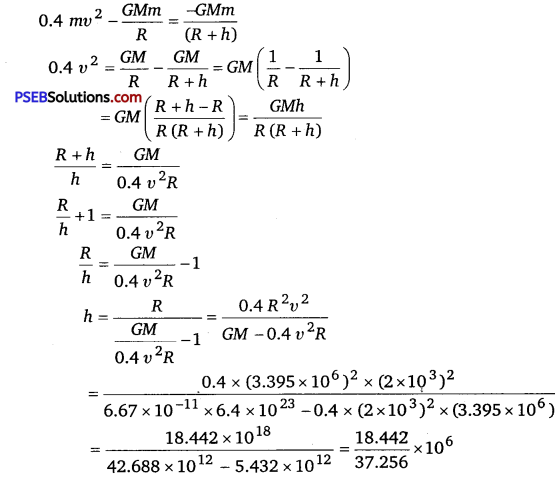= 495 x 103 m = 495 km